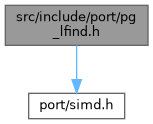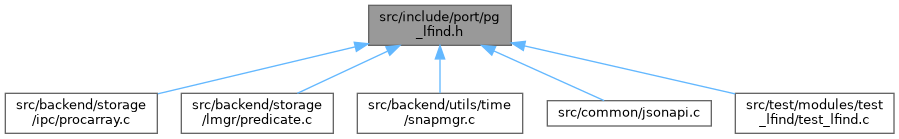PostgreSQL Source Code  git master
pg_lfind.h File Reference
`#include "port/simd.h"`
Include dependency graph for pg_lfind.h:This graph shows which files directly or indirectly include this file:Go to the source code of this file.

## Functions

static bool pg_lfind8 (uint8 key, uint8 *base, uint32 nelem)

static bool pg_lfind8_le (uint8 key, uint8 *base, uint32 nelem)

static bool pg_lfind32 (uint32 key, uint32 *base, uint32 nelem)

## ◆ pg_lfind32()

 static bool pg_lfind32 ( uint32 key, uint32 * base, uint32 nelem )
inlinestatic

Definition at line 90 of file pg_lfind.h.

91 {
92  uint32 i = 0;
93
94 #ifndef USE_NO_SIMD
95
96  /*
97  * For better instruction-level parallelism, each loop iteration operates
98  * on a block of four registers. Testing for SSE2 has showed this is ~40%
99  * faster than using a block of two registers.
100  */
101  const Vector32 keys = vector32_broadcast(key); /* load copies of key */
102  const uint32 nelem_per_vector = sizeof(Vector32) / sizeof(uint32);
103  const uint32 nelem_per_iteration = 4 * nelem_per_vector;
104
105  /* round down to multiple of elements per iteration */
106  const uint32 tail_idx = nelem & ~(nelem_per_iteration - 1);
107
108 #if defined(USE_ASSERT_CHECKING)
109  bool assert_result = false;
110
111  /* pre-compute the result for assert checking */
112  for (i = 0; i < nelem; i++)
113  {
114  if (key == base[i])
115  {
116  assert_result = true;
117  break;
118  }
119  }
120 #endif
121
122  for (i = 0; i < tail_idx; i += nelem_per_iteration)
123  {
124  Vector32 vals1,
125  vals2,
126  vals3,
127  vals4,
128  result1,
129  result2,
130  result3,
131  result4,
132  tmp1,
133  tmp2,
134  result;
135
136  /* load the next block into 4 registers */
139  vector32_load(&vals3, &base[i + nelem_per_vector * 2]);
140  vector32_load(&vals4, &base[i + nelem_per_vector * 3]);
141
142  /* compare each value to the key */
143  result1 = vector32_eq(keys, vals1);
144  result2 = vector32_eq(keys, vals2);
145  result3 = vector32_eq(keys, vals3);
146  result4 = vector32_eq(keys, vals4);
147
148  /* combine the results into a single variable */
149  tmp1 = vector32_or(result1, result2);
150  tmp2 = vector32_or(result3, result4);
151  result = vector32_or(tmp1, tmp2);
152
153  /* see if there was a match */
154  if (vector32_is_highbit_set(result))
155  {
156  Assert(assert_result == true);
157  return true;
158  }
159  }
160 #endif /* ! USE_NO_SIMD */
161
162  /* Process the remaining elements one at a time. */
163  for (; i < nelem; i++)
164  {
165  if (key == base[i])
166  {
167 #ifndef USE_NO_SIMD
168  Assert(assert_result == true);
169 #endif
170  return true;
171  }
172  }
173
174 #ifndef USE_NO_SIMD
175  Assert(assert_result == false);
176 #endif
177  return false;
178 }
unsigned int uint32
Definition: c.h:495
int i
Definition: isn.c:73
Assert(fmt[strlen(fmt) - 1] !='\n')

References Assert(), i, and sort-test::key.

Referenced by test_lfind32(), TransactionIdIsInProgress(), XidInMVCCSnapshot(), and XidIsConcurrent().

## ◆ pg_lfind8()

 static bool pg_lfind8 ( uint8 key, uint8 * base, uint32 nelem )
inlinestatic

Definition at line 26 of file pg_lfind.h.

27 {
28  uint32 i;
29
30  /* round down to multiple of vector length */
31  uint32 tail_idx = nelem & ~(sizeof(Vector8) - 1);
32  Vector8 chunk;
33
34  for (i = 0; i < tail_idx; i += sizeof(Vector8))
35  {
37  if (vector8_has(chunk, key))
38  return true;
39  }
40
41  /* Process the remaining elements one at a time. */
42  for (; i < nelem; i++)
43  {
44  if (key == base[i])
45  return true;
46  }
47
48  return false;
49 }
static void vector8_load(Vector8 *v, const uint8 *s)
Definition: simd.h:106
uint64 Vector8
Definition: simd.h:60
static bool vector8_has(const Vector8 v, const uint8 c)
Definition: simd.h:160

References i, sort-test::key, vector8_has(), and vector8_load().

Referenced by json_lex_string(), and test_lfind8_internal().

## ◆ pg_lfind8_le()

 static bool pg_lfind8_le ( uint8 key, uint8 * base, uint32 nelem )
inlinestatic

Definition at line 58 of file pg_lfind.h.

59 {
60  uint32 i;
61
62  /* round down to multiple of vector length */
63  uint32 tail_idx = nelem & ~(sizeof(Vector8) - 1);
64  Vector8 chunk;
65
66  for (i = 0; i < tail_idx; i += sizeof(Vector8))
67  {
69  if (vector8_has_le(chunk, key))
70  return true;
71  }
72
73  /* Process the remaining elements one at a time. */
74  for (; i < nelem; i++)
75  {
76  if (base[i] <= key)
77  return true;
78  }
79
80  return false;
81 }
static bool vector8_has_le(const Vector8 v, const uint8 c)
Definition: simd.h:211

References i, sort-test::key, vector8_has_le(), and vector8_load().

Referenced by json_lex_string(), and test_lfind8_le_internal().